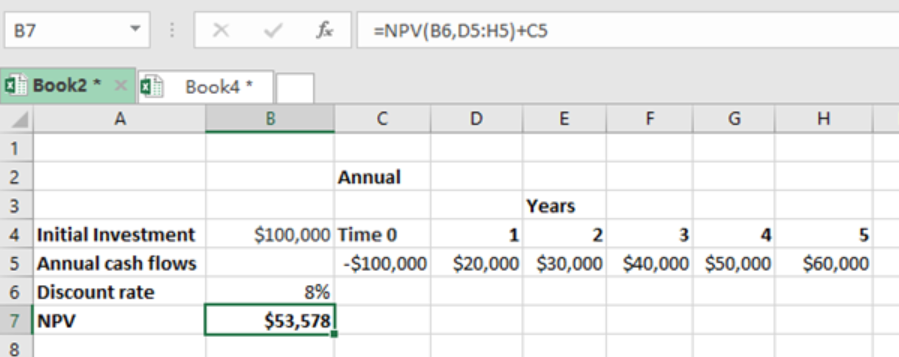Get instant live expert help with Excel or Google Sheets“My Excelchat expert helped me in less than 20 minutes, saving me what would have been 5 hours of work!”

#### Post your problem and you’ll get expert help in seconds.

Your message must be at least 40 characters
Our professional experts are available now. Your privacy is guaranteed.

# How Does the Net Present Value of Future Cash Flows Work in Excel?

The net present value, simply known as NPV, refers to the current worth of future cash flow. By a future cash flow we mean the amount of money that you will get in future by making an investment now.Figure 1

• Any competent employee of any financial institution must know how to value the company’s worth, whether present value or future value. This post looks at how to value a firm’s investment alternatives, with a view of knowing which investment opportunities are worth undertaking.
• Excel has many financial functions that are so important when it comes to valuation.
• One among the most important is the Excel NPV function, which looks at the net present value of future cash flows.

## How does NPV of future cash flow work in excel?

For you to get the net present value in excel, you first need to have a discount rate, future cash flows, and an initial investment. Note that excel assumes that the discount rate provided is in an annual form.

## General syntax of the formula

`=NPV(rate, future cash flows) + Initial investment`

• While calculating the net present value of a future cash flow, you need to first understand whether the future cash flows shall be discounted monthly, semi-annually, quarterly or monthly.
• This will affect both the periods supplied as well as the rate.
• In our example above, we have specified the initial investment, annual cash flows for five periods, and the discount rate.
• To calculate the present value of future cash flows, we have used the formula below;
`=NPV(B6,D5:H5)+C5`
• When we present Enter, we get \$53, 578 as the net present value.

## Instant Connection to an Expert through our Excelchat Service

Most of the time, the problem you will need to solve will be more complex than a simple application of a formula or function. If you want to save hours of research and frustration, try our live Excelchat service! Our Excel Experts are available 24/7 to answer any Excel question you may have. We guarantee a connection within 30 seconds and a customized solution within 20 minutes.

Solution examplesI have a figure which is the sum of 9 months of payments. Each payment decreases by the same amount (X) so the first payment is Y, second payment is Y-X, third is Y-(Xx2), forth is Y-(Xx3) and so on. I am trying to calculate what each of the 9 payments should be.
Solved by A. W. in 60 mins